# HSSlive: Plus One & Plus Two Notes & Solutions for Kerala State Board

## BSEB Class 10 Maths Chapter 3 Pair of Linear Equations in Two Variables Ex 3.2 Textbook Solutions PDF: Download Bihar Board STD 10th Maths Chapter 3 Pair of Linear Equations in Two Variables Ex 3.2 Book AnswersBSEB Class 10 Maths Chapter 3 Pair of Linear Equations in Two Variables Ex 3.2 Textbook Solutions PDF: Download Bihar Board STD 10th Maths Chapter 3 Pair of Linear Equations in Two Variables Ex 3.2 Book Answers

BSEB Class 10th Maths Chapter 3 Pair of Linear Equations in Two Variables Ex 3.2 Textbooks Solutions and answers for students are now available in pdf format. Bihar Board Class 10th Maths Chapter 3 Pair of Linear Equations in Two Variables Ex 3.2 Book answers and solutions are one of the most important study materials for any student. The Bihar Board Class 10th Maths Chapter 3 Pair of Linear Equations in Two Variables Ex 3.2 books are published by the Bihar Board Publishers. These Bihar Board Class 10th Maths Chapter 3 Pair of Linear Equations in Two Variables Ex 3.2 textbooks are prepared by a group of expert faculty members. Students can download these BSEB STD 10th Maths Chapter 3 Pair of Linear Equations in Two Variables Ex 3.2 book solutions pdf online from this page.

## Bihar Board Class 10th Maths Chapter 3 Pair of Linear Equations in Two Variables Ex 3.2 Books Solutions

 Board BSEB Materials Textbook Solutions/Guide Format DOC/PDF Class 10th Subject Maths Chapter 3 Pair of Linear Equations in Two Variables Ex 3.2 Chapters All Provider Hsslive

## How to download Bihar Board Class 10th Maths Chapter 3 Pair of Linear Equations in Two Variables Ex 3.2 Textbook Solutions Answers PDF Online?

2. Click on the Bihar Board Class 10th Maths Chapter 3 Pair of Linear Equations in Two Variables Ex 3.2 Answers.
3. Look for your Bihar Board STD 10th Maths Chapter 3 Pair of Linear Equations in Two Variables Ex 3.2 Textbooks PDF.
4. Now download or read the Bihar Board Class 10th Maths Chapter 3 Pair of Linear Equations in Two Variables Ex 3.2 Textbook Solutions for PDF Free.

## BSEB Class 10th Maths Chapter 3 Pair of Linear Equations in Two Variables Ex 3.2 Textbooks Solutions with Answer PDF Download

Find below the list of all BSEB Class 10th Maths Chapter 3 Pair of Linear Equations in Two Variables Ex 3.2 Textbook Solutions for PDF’s for you to download and prepare for the upcoming exams:

## BSEB Bihar Board Class 10th Maths Solutions Chapter 3 Pair of Linear Equations in Two Variables Ex 3.2

Question 1.
Form the pair of linear equations in the following problems, and find their solution graphically.

1. 10 students of Class X took part in a Mathematics quiz. If the number of girls is 4 more than the number of boys, If the number of girls is 4 more than the number of boys, find the number of boys and girls who took part in the quiz.

2. 5 pencils and 7 pens together cost Rs 50, whereas 7 pencils and 5 pens together cost Rs 46. Find the cost of one pencil and that of one pen.
Solution:
1. Let us denote the number of boys by x and the number of girls by y. Then, the equations formed are
x + y = 10 ………………. (1)
and y = x + 4 i.e., – x + y = 4 ……………… (2)
Let us draw the graphs of equations (1) and (2) by finding two solutions for each of these equations. The solutions of the equations are given in the tables: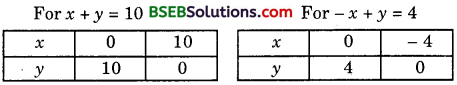Plot the points and draw the lines passing through them to represent the equations as shown.
These two lines intersect at (3, 7). So, x = 3 and y = 7 is the required solution.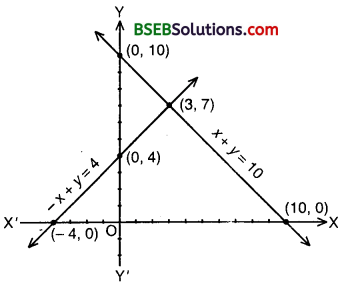Hence, the number of boys and girls are 3 and 7 respectively.

Verification:
Put x = 3 and y = 7 in (1) and (2), we find that both the equations are satisfied.

2. Let us denote the cost of one pencil by Rs x and one pen by Rs y. Then, the equations, formed are
5x + 7y = 50 ……………….. (1)
and 7x + 5y = 46 ………………… (2)
Let us draw the graphs of equations (1) and (2) by finding two solutions for each of these equations. The solutions of the equations are given in tables: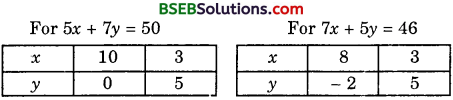Plot the points and draw the lines passing through them to represent the equations as shown.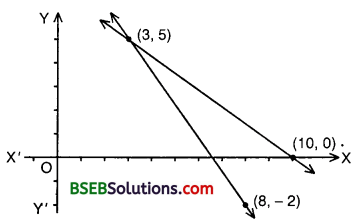These two lines intersect at (3, 5). So, x = 3 and y = 5 is the required solution.
Hence, the cost of one pencil is Rs 3 and that of one pen is Rs 5.

Verification:
Put x = 3 and y = 5 in (1) and (2), we find that both the equations are satisfied.

Question 2.
On comparing the ratios 𝑎1𝑎2, 𝑏1𝑏2 and 𝑐1𝑐2, find out whether the lines representing the following pairs
of linear equations intersect at a point, are parallel or coincide.

1. 5x – 4y + 8 = 0; 7x + 6y – 9 = 0
2. 9x + 3y + 12 = 0; 18x + 6y + 24 = 0
3. 6x – 3y + 10 = 0; 2x – y + 9 = 0

Solution:
1. The given pair of linear equations is
5x – 4y + 8 = 0 ……………… (1)
and 7x + 6y – 9 = 0 …………….. (2)
Here, 918 = 36 = 1224 [∵ Each = 12]
∴ lines (1) and (2) are coincident lines.

2. The given pair of linear equations is
9x + 3y + 12 = 0 ………………… (1)
and 18x + 6y + 24 = 0 ……………………… (2)
Here, 918 = 36 = 1224 [∵ Each = 12]
∴ lines (1) and (2) are coincident lines.

3. The given pair of linear equations is
6x – 3y + 10 = 0 ……………….. (1)
and 2x – y + 9 = 0 ……………… (2)
Here, 62 = −3−1 ≠ 109
∴ lines (1) and (2) are parallel lines.

Question 3.
On comparing the ratios 𝑎1𝑎2, 𝑏1𝑏2 and 𝑐1𝑐2, find out whether the following pairs of linear equations are consistent, or inconsistent.

1. 3x + 2y = 5; 2x – 3y = 7
2. 2x – 3y = 8; 4x – 6y = 9
3. 32x + 53y = 7; 9x – 10y = 14
4. 5x – 3y = 11; – 10x + 6y = – 22
5. 43x + 2y = 8; 2x + 3y = 12

Solution:
1. 3x + 2y = 5; 2x – 3y = 7. That is, they are:
3x + 2y – 5 = 0; 2x – 3y – 7 = 0
a1 = 3, b1 = 2, c1 = – 5; a2 = 2, b2 = – 3, c2 = – 7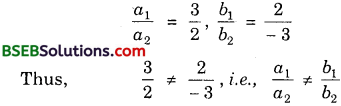∴ The pair of linear equations is consistent.

2. 2x – 3y = 8; 4x – 6y = 9. That is they are 2x – 3y – 8 = 0; 4x – 6y – 9 = 0
a1 = 2, b1 = – 3, c1 = – 8; a2 = 4, b2 = – 6, c2 = – 9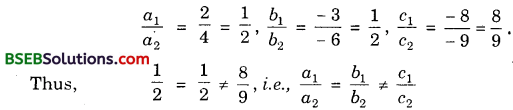∴ The pair of linear equations is consistent.

3. 32x + 53y = 7; 9x – 10y = 14. That is, they are 32x + 53y – 7 = 0 9x – 10y – 14 = 0
a1 = 32, b1 = 53, c1 = – 7; a2 = 9, b2 = – 10, c2 = – 14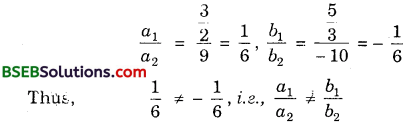∴ The pair of linear equations is consistent.

4. 5x – 3y = 11; – 10x + 6y = – 22. That is, they are:
5x – 3y – 11 = 0; – 10x + 6y + 22 = 0
a1 = 5, b1 = – 3, c1 = – 11; a2 = – 10, b2 = 6, c2 = 22∴ The pair of linear equations is consistent (and dependent).

5. 43x + 2y = 8; 2x + 3y = 12. That is, they are:
43x + 2y – 8 = 0; 2x + 3y – 12 = 0
a1 = 43, b1 = 2, c1 = – 8; a2 = 2, b2 = 3, c2 = – 12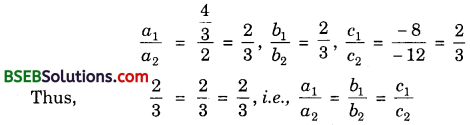∴ The pair of linear equations is consistent (and dependent).

Question 4.
Which of the following pairs of linear equations are consistent? Obtain the solution in such cases graphically.

1. x + y = 5, 2x + 2y = 10
2. x – y = 8, 3x – 3y = 16
3. 2x + y – 16 = 0, 4x – 2y – 4 = 0
4. 2x – 2y – 2 = 0, 4x – 4y – 5 = 0

Solution:
1. Graph of x + y = 5 :
We have: x + y = 5 or y = 5 – x
When x = 0, y = 5; when x = 5, y = 0.
Thus, we have the following table:Plot the points A(0, 5) and B(5, 0) on the graph paper, Join A and B and extend it on both sides to obtain the graph of x + y = 5.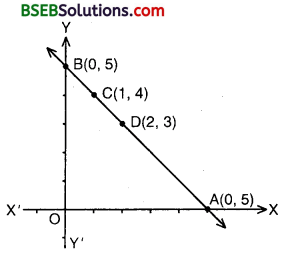Graph of 2x + 2y = 10:
We have: 2x + 2y = 10 or 2y = 10 – 2x
When x = 1, y = 5 – 1 = 4; when x = 2, y = 5 – 2 = 3
Thus, we have the following table: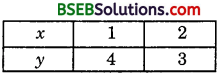Plotting the points C(1, 4) and D(2, 3) on the graph paper and drawing a line passing through these points on the same graph paper, we obtain the graph of 2x + 2y = 10. We find that C and D both lie on the graph of x + y = 5. Thus, the graphs of the two equations are coincident. Consequently, every solution of one equation is a solution of the other. Hence, the system of equations has infinitely many solutions, i.e., consistent and dependent.

2. Graph of x – y = 8:
We have: x – y = 8 or y = x – 8
When x = 0, y = – 8 when x = 8, y = 0
Thus, we have the following table: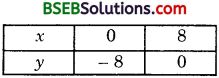Plot the points A(0, – 8) and B(8, 0) on a graph paper. Join A and B and extend it on both sides to obtain the graph of x – y = 8 as shown.
Graph of 3x – 3y = 16:
We have: 3x – 3y = 16 or 3y = 3x – 16 or y = 3𝑥−163
When x = 0, y = −163 = – 513;
When x = 163 = 513, y = 0
Thus, we have the following table: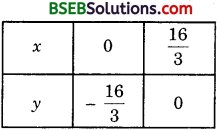Plot the points C(0, −163) and D(163, 0) on the same graph paper. Join C and D and extend it on both sides to obtain the graph of 3x – 3y = 16 as shown. We find the graphs of x – y = 8 and 3x – 3y = 16 are parallel. So, the two lines have no common point. Hence, the given equations has no solution, i.e., inconsistent.3. Graph of 2x + y – 6 = 0:
We have: 2x + y – 6 = 0 or y = 6 – 2x
When x = 0, y = 6 – 0 = 6; when x = 3, y = 6 – 6 = 0
Thus, we have the following table: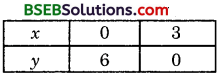Plot the points A(0, 6) and B(3, 0) on a graph paper. Join A and B and extend it on both sides to obtain the graph of 2x + y – 6 = 0 as shown.
Graph of 4x – 2y – 4 = 0:
We have: 4x – 2y – 4 = 0 or 2y = 4x – 4 or y = 2x – 2
When x = 0, y = – 2; when x – 1, y = 0
Thus, we have the following table: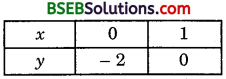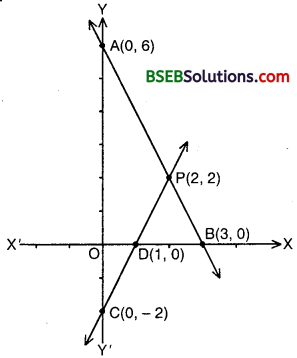Plotting the points C(0, – 2) and D(2, 0) on the same graph and drawing a line joining them as shown, we obtain the graph of 4x – 2y – 4 = 0.
Clearly, the two lines intersect at point P(2, 2).
Hence, x = 2, y = 2 is the solution of the given equations, i.e., consistent.

Verification:
Putting x = 2, y = 2 in the given equations, we find that both the equations are satisfied.

4. Graph of 2x – 2y – 2 = 0:
We have:
2x – 2y – 2 = 0 or 2y = 2x – 2 or y = x – 1
When x = 2, y = 0; when x = 0, y = – 2
Thus, we have the following table: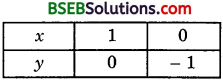Plot the points A(1, 0) and B(0, – 1) on a graph paper. Join A and B and extend it on both sides to obtain the graph of 2x – 2y – 2 = 0.
Graph of 4x – 4y – 5 = 0:
We have:
4x – 4y – 5 = 0 or 4y = 4x – 5 or y = 4𝑥−54
When x = 0, y = – 54; when x = 54, y = 0.
Thus, we have the following table:Plot the points C(0, −54) and D(54, 0) on the same graph paper. Join C and D and extend it on both sides to obtain the graph of 4x – 4y – 5 = 0 as shown. We find the graphs of these equations are parallel lines. So, the two lines have no common point. Hence, the given system of equations has no solution, i.e., inconsistent.

Question 5.
Half the perimeter of a rectangular garden, whose length is 4 m more than its width, is 36 m. Find the dimensions of the garden.
Solution:
Let the length of the garden be x m and its width be y m.
Then, perimeter = 2( Length + Width)
= 2(x + y)
Therefore, half perimeter = (x + y)
But it is given as 36
∴ (x + y) = 36 ………………. (1)
Also, x = y + 4 i.e; x – y = 4 ………………… (2)
For finding the solution of (1) and (2) graphically, we form the following tables: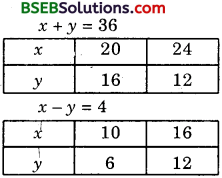Draw the graphs by joining points (20, 16) and (24, 12) and points (10, 6) and (16, 12). The two lines intersect at point (20, 16) as shown in the figure.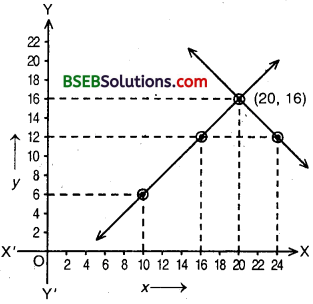Hence, Length = 20 m and width = 16 m.

Question 6.
Given the linear equation 2x + 3y – 8 = 0, write another linear equation in two variables such that the geometrical representation of the pair so formed is:

1. intersecting lines
2. parallel lines
3. coincident lines

Solution:
Given linear equation is 2x + 3y – 8 = 0 ……………… (1)
1. For intersecting lines, we know that
𝑎1𝑎2≠𝑏1𝑏2
Any intersecting line may be taken as
5x + 2y – 9 – 0

2. For parallel lines, 𝑎1𝑎2 = 𝑏1𝑏2 ≠ 𝑐1𝑐2
Any line parallel to (1) may be taken as
4x + 6y – 3 = 0

3. For coincident lines, 𝑎1𝑎2 = 𝑏1𝑏2 = 𝑐1𝑐2
Any line coincident to (1) may be taken as
6x + 9x – 24 = 0
Note: Answers can differ from person to person.

Question 7.
Draw the graphs of the equations x – y + 1 = 0 and 3x + 2y – 12 = 0. Determine the coordinates of the vertices of the triangle formed by these lines and the x – axis, and shade the triangular region.
Solution:
For the graph of x – y + 1 = 0:
We have:
x – y + 1 = 0 or y = x + 1
When x = 0, y = 1; when x = – 1, y’= 0.
Thus, we have the following table:Plot the points A(0, 1) and B(- 1, 0) on a graph paper. Join A and B and extend it on both sides to obtain the graph of x – y + 1 = 0.For the graph of 3x + 2y – 12 = 0:
We have:
3x + 2y – 12 = 0 or 2y = 12 – 3x or y = 12−3𝑥2
When x = 4, y = 12−122 = 0
When x = 0, y = 122 = 6
Thus, we have the following table: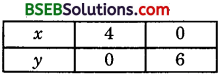Plot the points C(4, 0) and D(0, 6) on the same graph paper and draw a line passing through these two points to obtain the graph of equation 3x + 2y – 12 = 0. Clearly, we obtain a ∆ PBC formed by the given lines and the x-axis. The co-ordinates of the vertices are P(2, 3), B(- 1, 0) and C(4, 0). Triangular region is shaded as shown.

## Bihar Board Class 10th Maths Chapter 3 Pair of Linear Equations in Two Variables Ex 3.2 Textbooks for Exam Preparations

Bihar Board Class 10th Maths Chapter 3 Pair of Linear Equations in Two Variables Ex 3.2 Textbook Solutions can be of great help in your Bihar Board Class 10th Maths Chapter 3 Pair of Linear Equations in Two Variables Ex 3.2 exam preparation. The BSEB STD 10th Maths Chapter 3 Pair of Linear Equations in Two Variables Ex 3.2 Textbooks study material, used with the English medium textbooks, can help you complete the entire Class 10th Maths Chapter 3 Pair of Linear Equations in Two Variables Ex 3.2 Books State Board syllabus with maximum efficiency.

## FAQs Regarding Bihar Board Class 10th Maths Chapter 3 Pair of Linear Equations in Two Variables Ex 3.2 Textbook Solutions

#### How to get BSEB Class 10th Maths Chapter 3 Pair of Linear Equations in Two Variables Ex 3.2 Textbook Answers??

Students can download the Bihar Board Class 10 Maths Chapter 3 Pair of Linear Equations in Two Variables Ex 3.2 Answers PDF from the links provided above.

#### Can we get a Bihar Board Book PDF for all Classes?

Yes you can get Bihar Board Text Book PDF for all classes using the links provided in the above article.

## Important Terms

Bihar Board Class 10th Maths Chapter 3 Pair of Linear Equations in Two Variables Ex 3.2, BSEB Class 10th Maths Chapter 3 Pair of Linear Equations in Two Variables Ex 3.2 Textbooks, Bihar Board Class 10th Maths Chapter 3 Pair of Linear Equations in Two Variables Ex 3.2, Bihar Board Class 10th Maths Chapter 3 Pair of Linear Equations in Two Variables Ex 3.2 Textbook solutions, BSEB Class 10th Maths Chapter 3 Pair of Linear Equations in Two Variables Ex 3.2 Textbooks Solutions, Bihar Board STD 10th Maths Chapter 3 Pair of Linear Equations in Two Variables Ex 3.2, BSEB STD 10th Maths Chapter 3 Pair of Linear Equations in Two Variables Ex 3.2 Textbooks, Bihar Board STD 10th Maths Chapter 3 Pair of Linear Equations in Two Variables Ex 3.2, Bihar Board STD 10th Maths Chapter 3 Pair of Linear Equations in Two Variables Ex 3.2 Textbook solutions, BSEB STD 10th Maths Chapter 3 Pair of Linear Equations in Two Variables Ex 3.2 Textbooks Solutions,
Share: